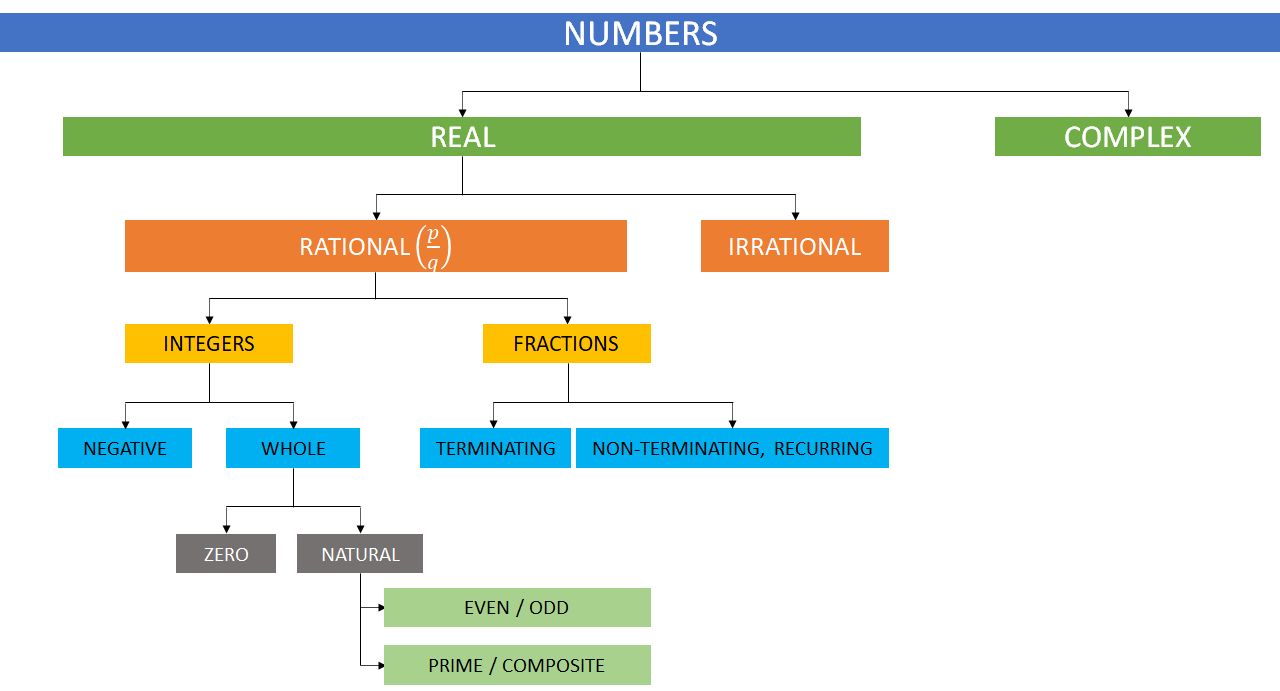# Concept: Classification

INTRODUCTION

Numbers is an extremely important topic for any MBA entrance exam. Apart from direct questions from this topic you will also find questions from other topics which require application of concepts from numbers.​​​​​​​

REAL & COMPLEX

As far as CAT and other MBA entrance exams is concerned, we will limit our discussion to only real numbers.

Real numbers are simply the combination of rational and irrational numbers, in the number system. In general, all the arithmetic operations can be performed on these numbers and they can be represented in the number line, also.

RATIONAL (Z) & IRRATIONAL

A rational number is a number that is in the form of p/q, where p and q are integers, and q is not equal to 0. Example: 1/3, 2/4, 1/5 etc.

A number which cannot be written in the form of p/q satisfying above criteria, is an irrational number. Example: π, √2, log2 etc.

FRACTIONS

A numerical quantity that is not a whole number. Example: 3/2, 0.5, 2/3 etc.

PROPER / IMPROPER

Remember:
• A fraction whose decimal value is between -1 and 1 is called a proper fraction
• A fraction whose decimal value is less than -1 or greater than 1 is called an improper fraction

Note: For a decimal to be a rational number, it should be:

• Either a terminating decimal. Example: 2.125, 0.5587 etc., or
• A non-terminating but recurring decimal. Example: $0.\overline{3}$ or $0.6\overline{25}$

CONVERTING DECIMAL TO FRACTION

Remember:

If x = $\mathrm{a}.\mathrm{b}\overline{\mathrm{c}}$, then

x =

INTEGERS

An integer is a number with no decimal or fractional part, from the set of negative and positive numbers, including zero. Examples of integers are: -5, 0, 1, 5,

NEGATIVE INTEGERS

Negative Integers are simply any integer with a value less than zero.

WHOLE NUMBERS (W)

Remember:
• The whole numbers are the numbers without fractions and it is a collection of positive integers and zero.
• They are also called non-negative integers.

NATURAL NUMBERS (N)

Remember:
• Counting numbers from 1 to infinity i.e., 1, 2, 3, and so on are called Natural Numbers.
• They are also called positive integers

PRIME / COMPOSITE

Remember:
• Prime numbers have exactly 2 factors. Example, 2, 3, 5, 7, 9 etc.
• 2 is the only even prime number.
• Any prime number greater than 3 can be written as (6k + 1) or (6k - 1)
• Composite numbers have more than 2 factors. Example: 4, 6, 10, 15 etc.
• 1 is neither prime nor composite

EVEN / ODD

Remember:
• Any number which is completely divisible by 2 is called an even number.
• 0 is an even number.

## Feedback

Help us build a Free and Comprehensive Preparation portal for various competitive exams by providing us your valuable feedback about Apti4All and how it can be improved.